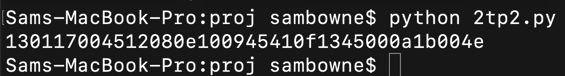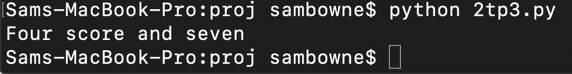# C 105: Two-Time Pad (15 pts)

## Background

One-time pads are very secure, but not if you use the pad more than once. Russia made this error during World War II and it led to complete compromise of their encryption system, as detailed in this book.

## What You Need

Any computer with Python 2.7.

Let's use this keystring:
```Unbreakable awesome secret secure system ```
To encrypt this message:
```Four score and seven ```
This Python code does the encryption:
```key = "Unbreakable awesome secret secure system" plaintext = "Four score and seven" i = 0 ciphertext = "" for p in plaintext: ip = ord(p) k = key[i] ik = ord(k) inew = ip ^ ik ciphertext += chr(inew) print p, hex(ip), k, hex(ik), hex(inew) i += 1 print ciphertext.encode("hex") ```
This program encrypts the string, as shown below.Here's a shorter program that does the same thing, with less output:

```key = "Unbreakable awesome secret secure system" plaintext = "Four score and seven" i = 0 ciphertext = "" for p in plaintext: ciphertext += chr( ord(p) ^ ord(key[i]) ) i += 1 print ciphertext.encode("hex") ```
This program encrypts the string, as shown below.Decrypting uses the same operation, since XOR reverses itself:

```key = "Unbreakable awesome secret secure system" ciphertext = "130117004512080e100945410f1345000a1b004e".decode("hex") i = 0 plaintext = "" for p in ciphertext: plaintext += chr( ord(p) ^ ord(key[i]) ) i += 1 print plaintext ```
This string, is decrypted, as shown below.## C 105.1: Using a Known Key (5 pts)

Use this key:
```Wow, what a great key! ```
Decrypt this ciphertext:
```0e20220c67383c413d7440 ```
The plaintext is the flag.

## C 105.2: Partial Key (10 pts)

The key starts with:
```The ```
Both these ciphertexts were encrypted with the same key:
```1a0d134503550e0a1d4542071f56 0d0710071d19490d0e5607004f00074e47 ```
To break this, start with a guess at the key, like this:
```The................................ ```
This shows the first three letters of the two plaintext messages, as shown below.From the partial plaintexts, you can guess another letter or two, and use those letters to extend the key. Continue adding one or two letters at a time until you get the whole plaintext.

The plaintext starting with "N" is the flag.

Posted 1-15-19
Format fixes 2-24-19
Ported to new scoring engine 7-11-19